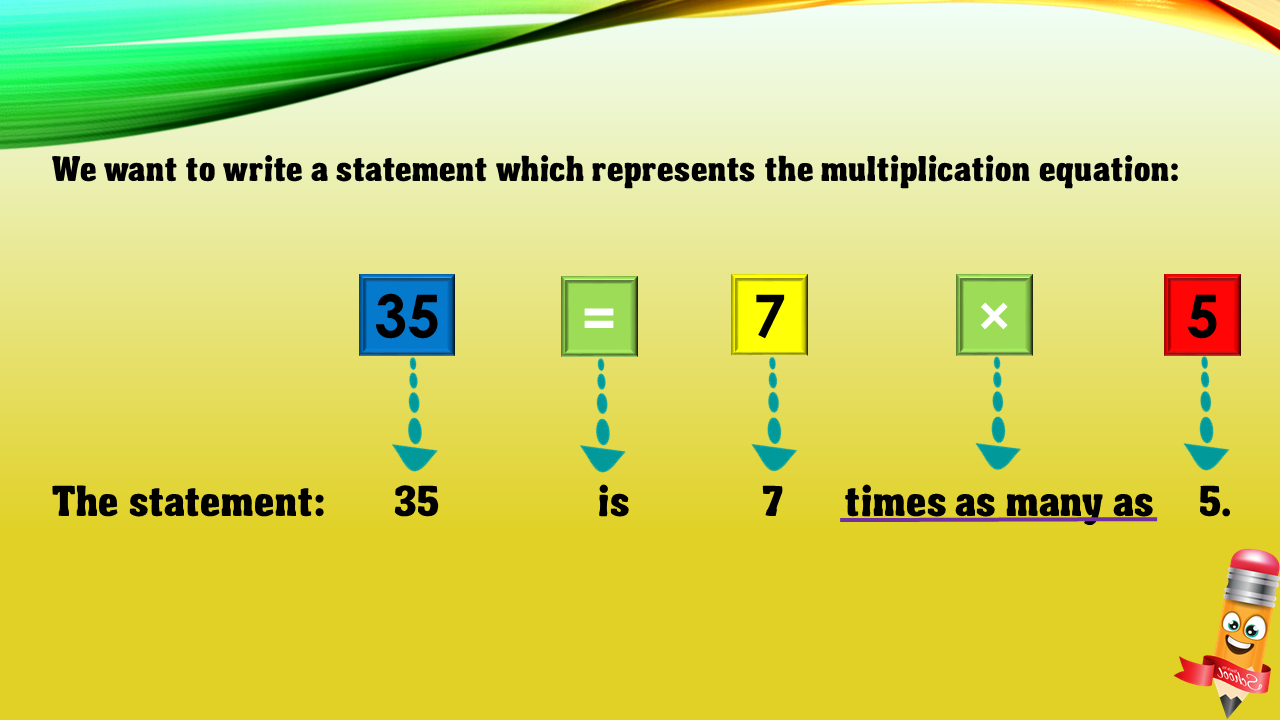1
visibility

Choose one statement which represents the equation: 35 = 7×5 ?

• A

35 times as many as 7 is 5.

• B

35 is 7 times as many as 5.

• C

35 is 7 more than 5.

• D

7 is 5 less than 35.